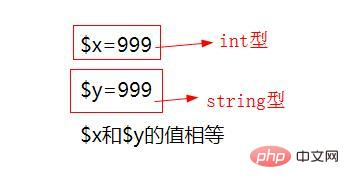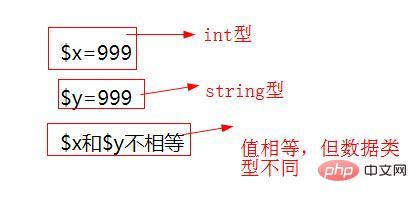# php中“==”符号的含义是什么```<?php header("content-type:text/html;charset=utf-8"); // 给变量赋整数值 \$x = 999; echo '\$x='.\$x."<br>"; // 给变量赋字符串值 \$y = '999'; echo '\$y='.\$y."<br>"; //比较\$x 和\$y if (\$x == \$y) echo '\$x和\$y的值相等'; else echo '\$x和\$y的值不相等'; ?>登录后复制``````<?php header("content-type:text/html;charset=utf-8"); // 给变量赋整数值 \$x = 999; echo '\$x='.\$x."<br>"; // 给变量赋字符串值 \$y = '999'; echo '\$y='.\$y."<br>"; //比较\$x 和\$y if (\$x === \$y) echo '\$x和\$y相等'; else echo '\$x和\$y不相等'; ?>登录后复制```PHP比较运算符

\$a == \$b等于如果类型转换后 \$a 和 \$b 的值相等，则返回 TRUE，否则返回 FALSE
\$a === \$b全等如果 \$a 和 \$b 不仅值相等，而且它们值的类型也相等，则返回 TRUE，否则返回 FALSE
\$a != \$b不等于如果类型转换后 \$a 的值不等于 \$b 的值，则返回TRUE，否则返回 FALSE
\$a <> \$b不等于与 != 相同，如果类型转换后 \$a 的值不等于 \$b 的值，则返回 TRUE，否则返回 FALSE
\$a !== \$b不全等如果 \$a 的值不等于 \$b的值，或者它们值的类型不同，则返回 TRUE，否则返回 FALSE
\$a < \$b小与如果 \$a 的值小于 \$b 的值，则返回 TRUE，否则返回 FALSE
\$a > \$b大于如果 \$a 的值大于 \$b 的值，则返回 TRUE，否则返回 FALSE
\$a <= \$b小于等于如果 \$a 的值小于或者等于 \$b 的值，则返回 TRUE，否则返回 FALSE
\$a >= \$b大于等于如果 \$a 的值大于或者等于 \$b 的值，则返回 TRUE，否则返回 FALSE
\$a <=> \$b太空船运算符（组合比较符）当 \$a小于、等于、大于 \$b时 分别返回一个小于、等于、大于0的整型值。 PHP7开始提供.
\$a ?? \$b ?? \$cNULL合并操作符从左往右第一个存在且不为 NULL 的操作数。如果都没有定义且不为 NULL，则返回 NULL。PHP7开始提供。